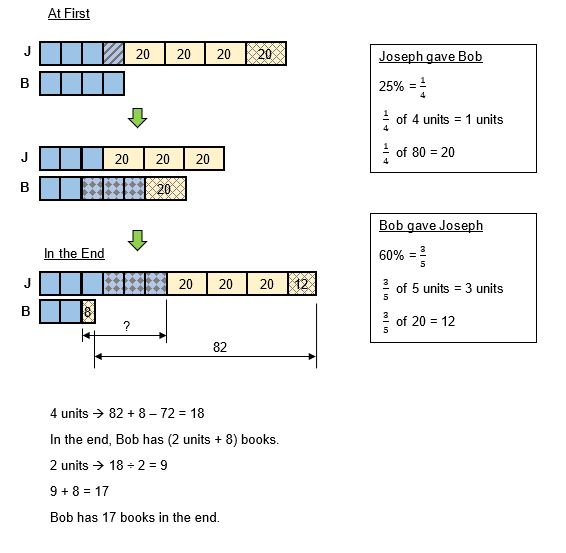# Question

Joseph has 80 more books than Bob. Joseph gave 25% of his books to Bob. In return, Bob gave 60% of his books to Joseph. In the end, Joseph had 82 more books than Bob. How many books does Bob have now?

my answer is 56. Am I correct? Thanks

My solution is as follows :

Joseph : 10 units + 80
Bob : 10 units

25% x (10 units + 80) = 2.5 units + 20

Joseph gave 25% of his books to Bob,
Joseph : 10u + 80 – 2.5 units – 20 = 7.5 units + 60
Bob : 10 units + 2.5 units + 20 = 12.5 units + 20

60% x (12.5 units + 20) = 7.5 units + 12

In return, Bob gave 60% of his books to Joseph,
Joseph : 7.5 units + 60 + 7.5 units + 12 = 15 units + 72
Bob : 12.5 units + 20 – 7.5 units – 12 = 5 units + 8

In the end, Joseph had 82 more books than Bob,
15 units + 72 – 5 units – 8 = 10 units + 64 = 82
1 unit = (82 – 64)/10 = 1.8
5 units + 8 = 5 x 1.8 + 8 = 17

Ans : 17 books.
(Note : This question is flawed.)

0 Replies 0 Likes

This is a multiple transfer question.

LCM (left)

75/100 × 4/10 = 300/1000

 Before T1 After T2 After Joseph 1000u + 80 3/4 750u + 60 1500u + 72 Bob 1000u 1250u + 20 4/10 500u + 8 Total 2000u + 80 2000u + 80 2000u + 80

1500u + 72 = 500u + 8 + 82

1500u – 500u = 90 – 72

1000u = 18

1u = 18 ÷ 1000 = 0.018

500u = 500 × 0.018 = 9

9 + 8 = 17

Note

There is a question error in this problem

Joseph before

1000u = 18

18 + 80 = 98

98 × 3/4 = 73.5 (not possible, you cannot tear a book)

0 Replies 0 Likes0 Replies 0 Likes